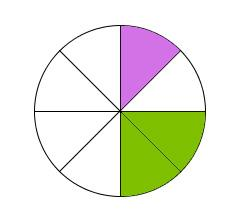# Difference 4307

I mean the number. If I reduce it by 0.5, then add 1/3, reduce the sum by 2/5 and increase the difference by 1/6, I get 0.3.

x =  0.7

### Step-by-step explanation:

x-0.5+1/3-2/5+1/6= 0.3

30x = 21

x = 21/30 = 0.7

x = 7/10 = 0.7

Our simple equation calculator calculates it.Did you find an error or inaccuracy? Feel free to write us. Thank you!

Tips for related online calculators
Need help calculating sum, simplifying, or multiplying fractions? Try our fraction calculator.
Do you have a linear equation or system of equations and looking for its solution? Or do you have a quadratic equation?